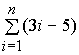Computer Science Foundation Exam

October 22 , 1999

Section I A

No Calculators!

Name: _______________________________

SSN: ________________________________

In this section of the exam, there are three (3) problems.

You must do all of them.

The weight of each problem in this section is indicated with the problem.

The algorithms in this exam are written in a combination of pseudocode, and programming language notation.

Partial credit can not be given unless all work is shown.

As always, be complete, yet concise, and above all be neat,

(1, 20%) Given the following array of numbers and algorithm, answer the questions below. Assume that the global array X[1..n] is correctly declared and contains the values shown.

 Array X 4 5 2 6 3 5 3 1 position 1 2 3 4 5 6 7 8

procedure R(a, b : integer)

j, k, y, z : integer;

y <- 0;
z <- 0;
j <- 1;
k <- b;
while (j <= k) do

if (X[k] < X[j]) then
y <- y + X[k];
X[k] <- X[k] + k;
k <- k - 1;
else
z <- z + X[j];
X[j] <- X[j] - j;
j <- j + 1;
endif
if (z > y) then
z <- z - 1; else
y <- y + 1; endif
endwhile
endprocedure

a) Show the array X after the procedure was called with R(5, 8)?

 Array X position 1 2 3 4 5 6 7 8

b) What value will the following variables contain after the while loop is finished?

 y z j k

(2, 18%)

The following are Postfix expressions. All values are single decimal digits and the

operations are addition "+", subtraction "–", multiplication "*" and division "/".

In each box below the Postfix expression, show ONLY the contents of the stack at the

indicated point in the Postfix string (point A, B or C). Put the final answer in the blank.

If the Postfix string is invalid, carry the operations as far as possible and write "invalid" as

a) 2 4 - 9 3 A+ + 5 3 - B / 7 4 - C * = _________________

## C

b) 7 2 3 4 * A - + 3 6 B 1 5 * - C + + = _________________

## C

Next to each Postfix expression, circle one answer to indicate if it is a valid Postfix string or not:

(no extra credit for providing the answer, if it is valid) (3 points each)

c) 3 4 + 2 4 * 4 7 - + 3 1 * - + Valid Invalid

d) 6 4 2 + * + 7 3 1 - + Valid Invalid

(3, 20%) Answer each of the following "timing" questions concerning an algorithm of a particular order and a data instance of a particular size. Assume that the run time is affected by the size of the data instance and not its composition.

a) For an O(2n) algorithm, an instance with n = 3 takes 16 seconds.

How long will it take with n = 5? ____________________

b) For an O(n2) algorithm, an instance with n = 5 takes 75 seconds.

If you used a different-sized data instance and it

took 48 seconds, how large must that instance be? ____________________

c) For an O(log2n) algorithm, a friend tells you that her instance took

48 seconds to run. You run the same program, on the same machine,

and your instance with n = 16 takes 32 seconds.

What size was her data set? ____________________

Given the following pseudocode segment, answer the questions below for an arbitrary n:

x <- 0
for i <- 1 to (2*n*n) do

for j <- 1 to (n-3) do
x <- x + 3

d) What is the Order of this code segment? ____________________

e) What will be the value of x when the for loops end? ____________________

Computer Science Foundation Exam

October 22 , 1999

Section I B

No Calculators!

Name: _______________________________

SSN: ________________________________

In this section of the exam, there are three (3) problems.

You must do all of them.

The weight of each problem in this section is indicated with the problem.

The algorithms in this exam are written in a combination of pseudocode, and programming language notation. The algorithms that you are asked to produce should use a syntax that is clear and unambiguous.

Partial credit can not be given unless all work is shown.

As always, be complete, yet concise, and above all be neat,

(4, 10%)

Given a global array of numbers X[1..n], you are to write a recursive algorithm that

will sum the values in the array. Assume that the array was already initialized and that the

SumArray function will be called as shown:

In the space below, write a RECURSIVE algorithm that correctly sums the array's contents.

SumArray(n)

(5, 20%) Find the closed form or exact value for the following:

( n is an arbitrary positive integer):

a)= _______________________

b)= ____________________

c) S = 4 + 5 + 6 + ... + (n-1) + n = _____________________

d) t(n) = t(n-1) + n, where t(0) = 2 ________________________

(6, 12%) Answer the following questions:

Suppose you have two algorithms that both correctly solve a problem.

One algorithm takes 2n steps and the other takes n3 steps. Assume that no other factors

(constants, the configuration of the data, etc.) affect the number of steps executed.

a) Which will take longer when n = 4 ?

the 2n steps algorithm
the n
3 steps algorithm
both take the same time

b) Which will take longer when n is very large?

the 2n steps algorithm
the n
3 steps algorithm
both take the same time

Name or describe one problem that is solvable in polynomial time:

c)

Name one problem that requires exponential time and is not in the class NP-Complete:

d)

Name two problems that are in the class of NP-Complete problems:

e)

f)